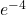## A 2020 kg car traveling at 14.2 m/s collides with a 2940 kg car that is initally at rest at a stoplight. The cars stick together and move 2.

Question

A 2020 kg car traveling at 14.2 m/s collides with a 2940 kg car that is initally at rest at a stoplight. The cars stick together and move 2.12 m before friction causes them to stop. Determine the coefficient of kinetic friction between the cars and the road, assuming that the negative acceleration is constant and all wheels on both cars lock at the time of impact.

in progress 0
5 months 2021-09-02T13:37:48+00:00 1 Answers 0 views 0

The answer is 3,064xExplanation:

When the collision happens, the momentum of the first car is applied to the both of them.

So we can calculate the force that acts on both cars as:

• The momentum of the first car is P = 2020 kg x 14.2 m/s = 28,684 kg.m/s
• The acceleration of both cars after the crash is going to be a = P / mtotal which will give us a = 28,684 / (2020+2940) = 5.78 m/s
• Since the second car was initially not moving, the final acceleration was calculated with the momentum of the first car.

Now we can find the force that acts on both of them by using the formula F = m.a which will give us the result as:

• F = (2020+2940) x 5.78 = 28,684

The friction force acts in the opposite direction and if they stop after moving 2.12 meters;

• Friction force is Ff = μ x N where μ is the friction coefficient and the N is the normal force which is (2020+2940) x 10 if we take gravitational force as 10, equals to 49,600.
• F – Ffriction = m x V
• 28,684 – μ x 49,600 = 4960 x 5.78
• μ = 3,064x